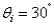# College Physics Reasoning and Relationships

Physics & Astronomy

## Quiz 24 :Geometrical OpticsLooking for Introductory Physics Homework Help?

## Quiz 24 :Geometrical Optics

Showing 1 - 20 of 127A line representing a wave front for a wave should be drawn:
Free
Multiple Choice

DA ray of light strikes a thick sheet of glass (n = 1.5) at an angle of 39° with the normal. Find the angle of the ray reflected off the glass surface with respect to the normal.
Free
Multiple Choice

DAs the angle of incidence is decreased for a ray incident on a reflecting surface, the angle between the incident and reflected rays ultimately approaches what value?
Free
Multiple Choice

DLight from a 560-nm monochromatic source is incident upon the surface of fused quartz (n = 1.56) at an angle of 30°. What is the angle of reflection from the surface?
Multiple ChoiceWhen viewing your image in a hand-held mirror, if you move the mirror away at a speed v, the image appears to:
Multiple ChoiceWhen light reflects and produces a clear image, this reflection is referred to as:
Multiple ChoiceTwo mirrors are joined together along a common side, the planes of the mirrors separated by the angle . If the joined mirrors can be used as a retroreflector when a beam of light strikes both surfaces (one after the other), what is the value of ?
Multiple ChoiceYou stand three feet away from a plane mirror. How far is it from you to your image?
Multiple ChoiceWhich of the following best describes the image from a plane mirror?
Multiple ChoiceWhen the reflection of an object is seen in a plane mirror, the image is:
Multiple ChoiceWhen the reflection of an object is seen in a plane mirror, the distance from the mirror to the image depends on:
Multiple ChoiceIf a man wishes to use a plane mirror on a wall to view both his head and his feet as he stands in front of the mirror, the required length of the mirror:
Multiple ChoiceHow large should a wall-mounted mirror be to view the upper half of one's height, h?
Multiple ChoiceA corner cube is formed by three plane mirrors perpendicular to one another resulting in a retroreflector. What is the angle between the directions of an incoming ray of light and of the corresponding outgoing ray of light for this device?
Multiple ChoiceTwo plane mirrors make an angle of 50° with one another. A ray of light parallel to mirror #1 reflects off mirror #2 and then strikes mirror #1. What is the angle of incidence the ray makes with mirror #1?
Multiple ChoiceA mirror lies flat on a table. A beam of light strikes the mirror with an angle of incidence or 15°, the reflected ray being to the right of the incident beam. The right edge of the mirror is then lifted so that the mirror now makes a 5° angle with the horizontal. What is the resulting angle between the incident beam and the reflected beam?
Multiple ChoiceThree flat layers of transparent material are stacked upon one another. The top layer has index of refraction n1, the middle has n2, and the bottom one has n3. If n1 < n2 < n3, at what angle of incidence will a ray of light traverse the three layers in a single straight line?
Multiple ChoiceThree flat layers of transparent material are stacked upon one another. The top layer has index of refraction n1, the middle has n2, and the bottom one has n3. If n1 > n2 > n3, and if a ray of light strikes the top layer at an angle of incidence, in which layer is the angle of refraction the greatest?
Multiple Choice